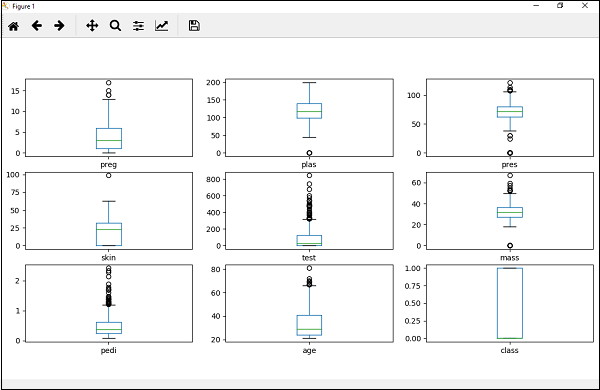# Machine Learning - Box and Whisker Plots

Box and Whisker plots, also called boxplots in short, is another useful technique to review the distribution of each attribute’s distribution. The following are the characteristics of this technique −

• It is univariate in nature and summarizes the distribution of each attribute.

• It draws a line for the middle value i.e. for median.

• It draws a box around the 25% and 75%.

• It also draws whiskers which will give us an idea about the spread of the data.

• The dots outside the whiskers signifies the outlier values. Outlier values would be 1.5 times greater than the size of the spread of the middle data.

## Example

In the following example, Python script will generate Density Plots for the distribution of attributes of Pima Indian Diabetes dataset.

from matplotlib import pyplot
path = r"C:\pima-indians-diabetes.csv"
names = ['preg', 'plas', 'pres', 'skin', 'test', 'mass', 'pedi', 'age', 'class']
data = read_csv(path, names = names)
data.plot(kind = 'box', subplots = True, layout = (3,3), sharex = False,sharey = False)
pyplot.show()


## OutputFrom the above plot of attribute’s distribution, it can be observed that age, test and skin appear skewed towards smaller values.

machine_learning_with_python_understanding_data_with_visualization.htm